#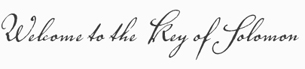Great Celestial ConjunctionThe most important diagram that Herschel has discovered originates from the Hebrew version of the Key of Solomon (Mafteah Shelomoh):Diagram A) Key of Solomon diagram appearing in a Hebrew version of the Key of Solomon

According to Herschel, the eight-pointed cross depicted at the 10 o’clock position in the left upper corner of the diagram is exactly the same token used in the diagram depicted below and supposedly represents the constellation Orion.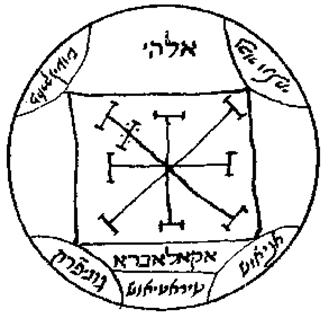Diagram B) According to Herschel, The eight-pointed cross in the diagram supposedly represents the constellation Orion.

Herschel claims that the symbolic representation of Orion is an eight-pointed cross. While the three belt stars certainly align and the four ‘corner’ stars can be connected to form diagonals, Herschel has been very creative to demonstrate how the six tiny stars that form the ‘Sword of Orion’ form yet the eighth radii of the eight-pointed cross. The problem is that there are no other known historic references that identified Orion with an eight-pointed cross.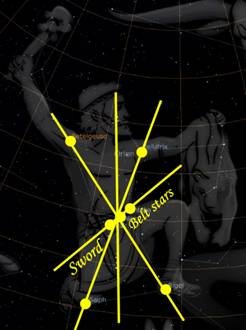Herschel’s rendition of the eight-pointed cross

About the square with the two semi circles in diagram A of the Hebrew Key of Solomon, Herschel writes the following on his website.

The meaning of the square and the two curves on each side of the star most likely represent sunrise and its path to sunset - the Ecliptic.

We fully support Herschel’s view that the two semicircles in between the two slopes represent the Sun. The glyph in the centre of diagram A looks very familiar with the Egyptian Akhet glyph where the Sun was depicted in between similar slopes. The Akhet glyph represented the rising and setting Sun on the horizon, and in hieroglyphic texts it is often translated as ‘horizon’.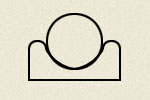Akhet

The Sun on the right represents the rising Sun in the East while the Sun on the left represents the setting Sun in the West. Only twice does the Sun rise and set exactly at the cardinal directions East and West, and this is during the Equinox. We therefore surmise that the two semicircles in the square of diagram A symbolise the Equinox. Following the same reasoning, the semicircles in diagram B should also represent the Sun. Wayne fails to give any reasonable explanation as to why these semicircles occur around a symbol that represents Orion. The semicircles representing the Sun at the corners of the square in the South and North region of the circle (globe) and its diagonals that form a cross could mean either one of two possible things:

• It represents the Solstices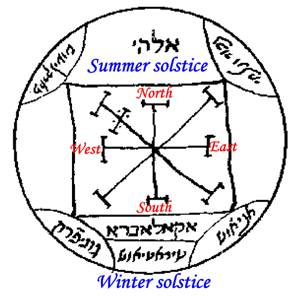Solstices

Notice that the diagonal in the upper left corner has two instead of one cross bar through its axis. This diagonal seems to be special and is associated with the Summer Solstice since its sunset occurs in the North-West, the region where the double cross bars occur. The axis connecting the cardinal directions East and West represents the Equinox axis.

The same double cross bars have been used in the eight pointed cross in diagram A. This time however the double cross bars occur at the axis representing the Equinox. This corresponds with the central depiction of the Equinox Sun in diagram A. We therefore suggest that diagram A is referring to the Equinox.Square with semicircles represents the Equinox as does the eight-pointed cross with double cross bars through the East-West axis. (encircled in red)

Considering this fact, the eight-pointed cross could also mean:

• The Sun at the Galactic Cross in the precession cycle.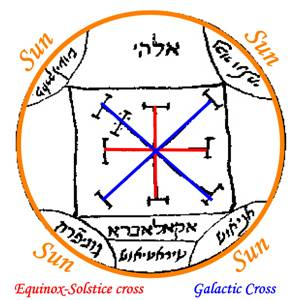Great Celestial Conjunction

The blue cross represents the Galactic Cross while the red cross represents the equinox-solstice axis or Earth Cross. The orange circle represents the precession cycle. During a Great Year, the Sun resides exactly four times on the Galactic Equator, twice at an Equinox and twice at a Solstice. Put in other words, during one revolution of the Earth Cross against the backdrop of the fixed Galactic Cross, the crosses overlap exactly four times. The symbology of the square with its four corners and its four suns attached to these corners could therefore represent these four Great Celestial Conjunctions of a Great Year.

Read more: Part II | Tetragrammaton | Great Celestial Conjunction | Pleiades starmap